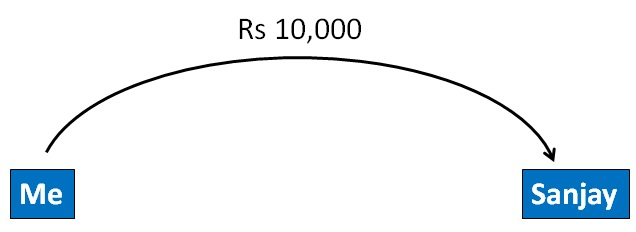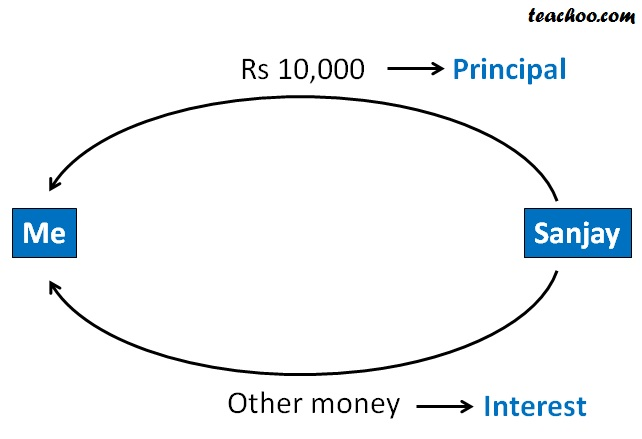Simple interest

Chapter 7 Class 7 Comparing Quantities
Concept wise

Interest is extra money paid for borrowing money.

If I gave Rs 10,000 to Sanjay for 1 year. I will take my

Rs 10,000….. and also some other money

(for letting him use my money for a period of time)After one year,#### Suppose I say, I give Rs 10,000 to Sanjay at 10% per annum interest How much amount does Sanjay have to pay after 1, 2, 3, 4, 5 years?

Interest = 10% per year

Interest will be on Principal

Interest for 1 year = 10% of Principal

= 10% × Principal

= 10% × 10,000

= 10/100 × 10,000

= 1/10 × 10,000

= 1,000

∴ Rs 1,000 interest is there after 1 year.

So, Total Amount = Principal + Interest

= 10,000 + 1,000

= 11,000

Interest for 2 years = 2 × Interest for 1 year

= 2 × 1000

= 2000

Let’s make a table

 Year Principal Interest Amount 1 st year 10,000 1000 11,000 2 nd year 10,000 2 × 1000 = 2000 12,000 3 rd year 10,000 3 × 1000 = 3000 13,000 4 th year 10,000 4 × 1000 = 4000 14,000 5 th year 10,000 5 × 1000 = 5000 15,000

So, Total amount after 2nd year = Principal + Interest

= 10,000 + 2,000

= 12,000

#### Suppose I have Rs 1,000 and I put it in the bank. What will be the amount I have after 1, 2, 3, 4, 5 years, if rate of interest is 5% p.a?

After 1 year,

Interest after 1st year = 5% of principal

= 5% of Rs 1000

= 5% × 1000

= 5/100 × 1000

= 5 × 10

= Rs 50

After 2 years,

Interest after 2nd year = 2 × Interest for 1st year

= 2 × Rs 50

= Rs 100

After 3 years,

Interest after 3rd year = 3 × Interest for 1st year

= 3 × Rs 50

= Rs 150

After 4 years,

Interest after 4th  year = 4 × Interest for 1st year

= 4 × Rs 50

= Rs 200

After 5 years,

Interest after 5th  year = 5 × Interest for 1st year

= 5 × Rs 50

= Rs 250

 Year Principal Interest Amount 1 st year 10,000 Rs 50 Rs 1050 2 nd year 10,000 RS 100 Rs 1100 3 rd year 10,000 Rs 150 Rs 1150 4 th year 10,000 Rs 200 Rs 1200 5 th year 10,000 Rs 250 Rs 1250

For finding Interest,

We use the formula

Simple Interest = (Principal × Rate × Time)/100

SI = PRT/100

#### For Rs 10,000 at 10% per annum. What will be the interest after 4 years?

P = Rs 10,000

R = 10%

T = 4

SI = (P × R × T)/100

= (10,000 × 10 × 4)/100

= 1000 × 4

= Rs 4000

So, Interest after 4 years is  Rs 4000

#### Suppose I have  Rs 1000 and I put it in the bank. What will be the amount I have after 5 years if Interest is 5% per annum?

P = Rs 1000

R = 5% p.a

T = 5 years

SI = (P × R × T)/100

= (1000 × 5 × 5)/100

= 10 × 5 × 5

= 10 × 25

= Rs 250

Amount = P + SI

= 1000 + 250

= Rs 1250

Learn in your speed, with individual attention - Teachoo Maths 1-on-1 Class